# Difference between revisions of "Poisson process"

2010 Mathematics Subject Classification: Primary: 60G55 Secondary: 60K25 [MSN][ZBL]

A stochastic processwith independent increments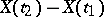,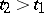, having a Poisson distribution. In the homogeneous Poisson process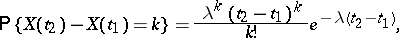(1)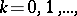for any. The coefficient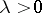is called the intensity of the Poisson process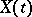. The trajectories of the Poisson processare step-functions with jumps of height 1. The jump points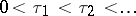form an elementary flow describing the demand flow in many queueing systems. The distributions of the random variables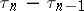are independent forand have exponential density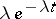,.

One of the properties of a Poisson process is that the conditional distribution of the jump points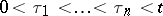when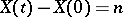is the same as the distribution of the variational series ofindependent samples with uniform distribution on. On the other hand, ifis the variational series described above, then as,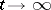andone obtains in the limit the distribution of the jumps of the Poisson process.

In an inhomogeneous process the intensity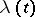depends on the timeand the distribution ofis defined by the formula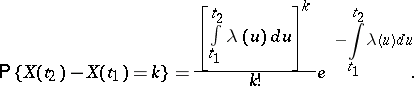Under certain conditions a Poisson process can be shown to be the limit of the sum of a number of independent "sparse" flows of fairly general form as this number increases to infinity. For certain paradoxes which have been obtained in connection with Poisson processes see [F].

How to Cite This Entry:
Poisson process. Encyclopedia of Mathematics. URL: http://encyclopediaofmath.org/index.php?title=Poisson_process&oldid=23169
This article was adapted from an original article by B.A. Sevast'yanov (originator), which appeared in Encyclopedia of Mathematics - ISBN 1402006098. See original article